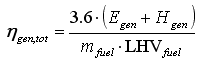# HOMER Pro 3.9

 Navigation: Glossary Generator Average Total EfficiencyType: Output Variable Units: % Symbol: ηgen,tot

This is the average total efficiency of the generator over the year, defined as the electrical plus thermal energy out divided by fuel energy in. HOMER uses the following equation to calculate the average total efficiency:where: Egen = the generator's total annual electrical production [kWh/yr] Egen = the generator's total annual thermal production [kWh/yr] mfuel = the generator's total annual fuel consumption [kg/yr] LHVfuel = the lower heating value of the fuel [MJ/kg]

The factor of 3.6 in the above equation arises because 1 kWh = 3.6 MJ.